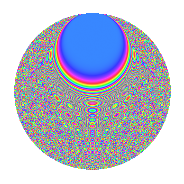# Properties

 Label 3024.2.ggLevel 3024 Weight 2 Character orbit gg Rep. character $$\chi_{3024}(257,\cdot)$$ Character field $$\Q(\zeta_{18})$$ Dimension 852 Sturm bound 1152

# Related objects

## Defining parameters

 Level: $$N$$ = $$3024 = 2^{4} \cdot 3^{3} \cdot 7$$ Weight: $$k$$ = $$2$$ Character orbit: $$[\chi]$$ = 3024.gg (of order $$18$$ and degree $$6$$) Character conductor: $$\operatorname{cond}(\chi)$$ = $$189$$ Character field: $$\Q(\zeta_{18})$$ Sturm bound: $$1152$$

## Dimensions

The following table gives the dimensions of various subspaces of $$M_{2}(3024, [\chi])$$.

Total New Old
Modular forms 3528 876 2652
Cusp forms 3384 852 2532
Eisenstein series 144 24 120

## Trace form

 $$852q + 9q^{3} - 9q^{5} + 6q^{7} - 3q^{9} + O(q^{10})$$ $$852q + 9q^{3} - 9q^{5} + 6q^{7} - 3q^{9} + 3q^{11} + 12q^{15} - 18q^{17} - 6q^{21} + 3q^{23} - 3q^{25} - 24q^{29} + 9q^{31} - 9q^{33} + 9q^{35} + 3q^{37} - 69q^{39} + 12q^{43} - 9q^{45} + 9q^{47} - 6q^{49} + 39q^{51} - 3q^{57} + 9q^{59} - 9q^{61} + 36q^{63} - 27q^{65} + 3q^{67} - 72q^{69} + 18q^{71} - 9q^{73} + 9q^{75} + 18q^{77} + 3q^{79} + 21q^{81} + 90q^{83} - 27q^{85} + 9q^{87} - 18q^{89} + 3q^{91} - 3q^{93} - 3q^{95} + 120q^{99} + O(q^{100})$$

## Decomposition of $$S_{2}^{\mathrm{new}}(3024, [\chi])$$ into newform subspaces

The newforms in this space have not yet been added to the LMFDB.

## Decomposition of $$S_{2}^{\mathrm{old}}(3024, [\chi])$$ into lower level spaces

$$S_{2}^{\mathrm{old}}(3024, [\chi]) \cong$$ $$S_{2}^{\mathrm{new}}(189, [\chi])$$$$^{\oplus 5}$$$$\oplus$$$$S_{2}^{\mathrm{new}}(378, [\chi])$$$$^{\oplus 4}$$$$\oplus$$$$S_{2}^{\mathrm{new}}(756, [\chi])$$$$^{\oplus 3}$$$$\oplus$$$$S_{2}^{\mathrm{new}}(1512, [\chi])$$$$^{\oplus 2}$$

## Hecke Characteristic Polynomials

There are no characteristic polynomials of Hecke operators in the database